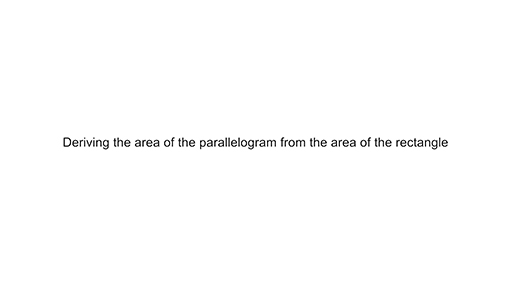### Become an OU studentTeaching mathematics

Start this free course now. Just create an account and sign in. Enrol and complete the course for a free statement of participation or digital badge if available.

# Using the area formula for a rectangle to derive other area formulas

The area formulas for parallelograms and triangles can be easily derived from the area formula of a rectangle (see Video 1).

Watch this video to see how the area formula of a rectangle can be used to derive the area of a parallelogram and then a triangle.

Download this video clip.Video player: Video 1 Deriving the area of a parallelogram and the area of a triangleVideo 1 Deriving the area of a parallelogram and the area of a triangle
Interactive feature not available in single page view (see it in standard view).

Since the parallelogram has been made by moving parts of the original rectangle without losing anything, the area of the parallelogram must be the same as the area of the rectangle.

It is worth bearing in mind that if the area formulas are promoted too quickly, before learners have conceptualised area as being a measure of the inside of a shape, then area measurement becomes a calculation without reference to the geometric situation.